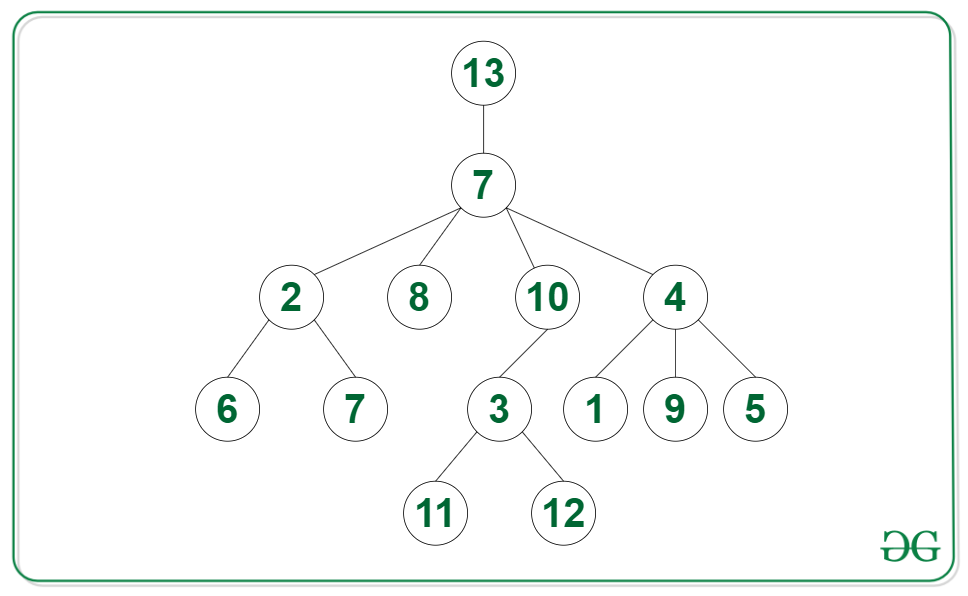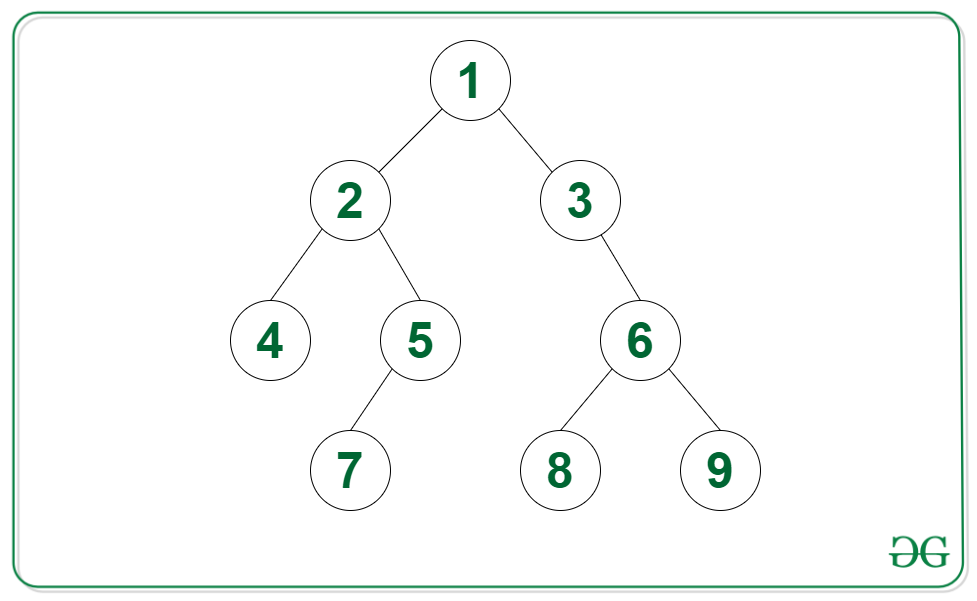Open in App
Not now

# Count of nodes in given N-ary tree such that their subtree is a Binary Tree

• Last Updated : 03 Dec, 2021

Given an N-ary tree root, the task is to find the count of nodes such that their subtree is a binary tree.

Example:

Input: Tree in the image belowOutput: 11
Explanation: The nodes such that there subtree is a binary tree are {2, 8, 10, 6, 7, 3, 1, 9, 5, 11, 12}.

Input: Tree in the image belowOutput: 9

Approach: The given problem can be solved by using the post-order traversal. The idea is to use recursion and check if the current node contains at most 2 children and if the children are valid binary trees. Below steps can be followed to solve the problem:

• Apply post-order traversal on the N-ary tree:
• Add the returned values of every child node to calculate the number of binary trees found at that node and store it in sum
• If the root has at most two children which are valid binary trees then the root is also a binary tree, so return a pair of sum + 1 and 1 to indicate a valid binary tree
• If the root has more than two child nodes or any of the children are not valid binary trees, then return the pair of sum and 0 to indicate an invalid binary tree
• Return the value at first index sum, from the pair returned from the post-order traversal.

Below is the implementation of the above approach:

## C++

 `// C++ code for the above approach``#include ``using` `namespace` `std;` `class` `Node``{``public``:``    ``vector children;``    ``int` `val;` `    ``// constructor``    ``Node(``int` `v)``    ``{` `        ``val = v;``        ``children = {};``    ``}``};``// Post-order traversal to find``// depth of all branches of every``// node of the tree``vector<``int``> postOrder(Node *root)``{` `    ``// Initialize a variable sum to``    ``// count number of binary trees``    ``int` `sum = 0;` `    ``// Integer to indicate if the tree``    ``// rooted at current root is a``    ``// valid binary tree``    ``int` `valid = 1;` `    ``// Use recursion on all child nodes``    ``for` `(Node *child : root->children)``    ``{` `        ``// Get the number of binary trees``        ``vector<``int``> binTrees = postOrder(child);` `        ``// If tree rooted at current child``        ``// is not a valid binary tree then``        ``// tree rooted at current root is``        ``// also not a valid binary tree``        ``if` `(binTrees == 0)``            ``valid = 0;` `        ``// If branches are unbalanced``        ``// then store -1 in height``        ``sum += binTrees;``    ``}` `    ``// Children are valid binary trees``    ``// and the number of children``    ``// are less than 3``    ``if` `(valid == 1 && root->children.size() < 3)``    ``{` `        ``// Root is also a valid binary tree``        ``sum++;``    ``}` `    ``// Children are leaf nodes but number``    ``// of children are greater than 2``    ``else``        ``valid = 0;` `    ``// Return the answer``    ``return` `{sum, valid};``}` `// Function to find the number of``// binary trees in an N-ary tree``int` `binTreesGeneric(Node *root)``{` `    ``// Base case``    ``if` `(root == NULL)``        ``return` `0;` `    ``// Apply post-order traversal on``    ``// the root and return the answer``    ``return` `postOrder(root);``}` `// Driver code``int` `main()``{` `    ``// Initialize the graph``    ``Node *twenty = ``new` `Node(20);``    ``Node *seven = ``new` `Node(7);``    ``Node *seven2 = ``new` `Node(7);``    ``Node *five = ``new` `Node(5);``    ``Node *four = ``new` `Node(4);``    ``Node *nine = ``new` `Node(9);``    ``Node *one = ``new` `Node(1);``    ``Node *two = ``new` `Node(2);``    ``Node *six = ``new` `Node(6);``    ``Node *eight = ``new` `Node(8);``    ``Node *ten = ``new` `Node(10);``    ``Node *three = ``new` `Node(3);``    ``Node *mfour = ``new` `Node(11);``    ``Node *zero = ``new` `Node(12);``    ``three->children.push_back(mfour);``    ``three->children.push_back(zero);``    ``ten->children.push_back(three);``    ``two->children.push_back(six);``    ``two->children.push_back(seven2);``    ``four->children.push_back(nine);``    ``four->children.push_back(one);``    ``four->children.push_back(five);``    ``seven->children.push_back(ten);``    ``seven->children.push_back(two);``    ``seven->children.push_back(eight);``    ``seven->children.push_back(four);``    ``twenty->children.push_back(seven);` `    ``// Call the function``    ``// and print the result``    ``cout << (binTreesGeneric(twenty));``}` `// This code is contributed by Potta Lokesh`

## Java

 `// Java implementation for the above approach` `import` `java.io.*;``import` `java.util.*;` `class` `GFG {` `    ``static` `class` `Node {` `        ``List children;``        ``int` `val;` `        ``// constructor``        ``public` `Node(``int` `val)``        ``{` `            ``this``.val = val;``            ``children = ``new` `ArrayList<>();``        ``}``    ``}` `    ``// Function to find the number of``    ``// binary trees in an N-ary tree``    ``public` `static` `int` `binTreesGeneric(Node root)``    ``{` `        ``// Base case``        ``if` `(root == ``null``)``            ``return` `0``;` `        ``// Apply post-order traversal on``        ``// the root and return the answer``        ``return` `postOrder(root)[``0``];``    ``}` `    ``// Post-order traversal to find``    ``// depth of all branches of every``    ``// node of the tree``    ``public` `static` `int``[] postOrder(Node root)``    ``{` `        ``// Initialize a variable sum to``        ``// count number of binary trees``        ``int` `sum = ``0``;` `        ``// Integer to indicate if the tree``        ``// rooted at current root is a``        ``// valid binary tree``        ``int` `valid = ``1``;` `        ``// Use recursion on all child nodes``        ``for` `(Node child : root.children) {` `            ``// Get the number of binary trees``            ``int``[] binTrees = postOrder(child);` `            ``// If tree rooted at current child``            ``// is not a valid binary tree then``            ``// tree rooted at current root is``            ``// also not a valid binary tree``            ``if` `(binTrees[``1``] == ``0``)``                ``valid = ``0``;` `            ``// If branches are unbalanced``            ``// then store -1 in height``            ``sum += binTrees[``0``];``        ``}` `        ``// Children are valid binary trees``        ``// and the number of children``        ``// are less than 3``        ``if` `(valid == ``1` `&& root.children.size() < ``3``) {` `            ``// Root is also a valid binary tree``            ``sum++;``        ``}` `        ``// Children are leaf nodes but number``        ``// of children are greater than 2``        ``else``            ``valid = ``0``;` `        ``// Return the answer``        ``return` `new` `int``[] { sum, valid };``    ``}` `    ``// Driver code``    ``public` `static` `void` `main(String[] args)``    ``{` `        ``// Initialize the graph``        ``Node twenty = ``new` `Node(``20``);``        ``Node seven = ``new` `Node(``7``);``        ``Node seven2 = ``new` `Node(``7``);``        ``Node five = ``new` `Node(``5``);``        ``Node four = ``new` `Node(``4``);``        ``Node nine = ``new` `Node(``9``);``        ``Node one = ``new` `Node(``1``);``        ``Node two = ``new` `Node(``2``);``        ``Node six = ``new` `Node(``6``);``        ``Node eight = ``new` `Node(``8``);``        ``Node ten = ``new` `Node(``10``);``        ``Node three = ``new` `Node(``3``);``        ``Node mfour = ``new` `Node(``11``);``        ``Node zero = ``new` `Node(``12``);``        ``three.children.add(mfour);``        ``three.children.add(zero);``        ``ten.children.add(three);``        ``two.children.add(six);``        ``two.children.add(seven2);``        ``four.children.add(nine);``        ``four.children.add(one);``        ``four.children.add(five);``        ``seven.children.add(ten);``        ``seven.children.add(two);``        ``seven.children.add(eight);``        ``seven.children.add(four);``        ``twenty.children.add(seven);` `        ``// Call the function``        ``// and print the result``        ``System.out.println(``            ``binTreesGeneric(twenty));``    ``}``}`

## Python3

 `# Python code for the above approach``class` `Node:` `  ``# constructor``  ``def` `__init__(``self``, v):``    ``self``.val ``=` `v;``    ``self``.children ``=` `[];` `# Post-order traversal to find``# depth of all branches of every``# node of the tree``def` `postOrder(root):` `  ``# Initialize a variable sum to``  ``# count number of binary trees``  ``sum` `=` `0``;` `  ``# Integer to indicate if the tree``  ``# rooted at current root is a``  ``# valid binary tree``  ``valid ``=` `1``;` `  ``# Use recursion on all child nodes``  ``for` `child ``in` `root.children:` `    ``# Get the number of binary trees``    ``binTrees ``=` `postOrder(child);` `    ``# If tree rooted at current child``    ``# is not a valid binary tree then``    ``# tree rooted at current root is``    ``# also not a valid binary tree``    ``if` `(binTrees[``1``] ``=``=` `0``):``      ``valid ``=` `0``;` `    ``# If branches are unbalanced``    ``# then store -1 in height``    ``sum` `+``=` `binTrees[``0``];``  `  `  ``# Children are valid binary trees``  ``# and the number of children``  ``# are less than 3``  ``if` `(valid ``=``=` `1` `and` `len``(root.children) < ``3``):` `    ``# Root is also a valid binary tree``    ``sum` `+``=` `1``  `  `  ``# Children are leaf nodes but number``  ``# of children are greater than 2``  ``else``:``    ``valid ``=` `0``;` `  ``# Return the answer``  ``return` `[ ``sum``, valid ];`  `# Function to find the number of``# binary trees in an N-ary tree``def` `binTreesGeneric(root):` `  ``# Base case``  ``if` `(root ``=``=` `None``):``    ``return` `0``;` `  ``# Apply post-order traversal on``  ``# the root and return the answer``  ``return` `postOrder(root)[``0``];`  `# Driver code` `# Initialize the graph``twenty ``=` `Node(``20``);``seven ``=` `Node(``7``);``seven2 ``=` `Node(``7``);``five ``=` `Node(``5``);``four ``=` `Node(``4``);``nine ``=` `Node(``9``);``one ``=` `Node(``1``);``two ``=` `Node(``2``);``six ``=` `Node(``6``);``eight ``=` `Node(``8``);``ten ``=` `Node(``10``);``three ``=` `Node(``3``);``mfour ``=` `Node(``11``);``zero ``=` `Node(``12``);``three.children.append(mfour);``three.children.append(zero);``ten.children.append(three);``two.children.append(six);``two.children.append(seven2);``four.children.append(nine);``four.children.append(one);``four.children.append(five);``seven.children.append(ten);``seven.children.append(two);``seven.children.append(eight);``seven.children.append(four);``twenty.children.append(seven);` `# Call the function``# and print the result``print``((binTreesGeneric(twenty)));` `# This code is contributed by gfgking`

## Javascript

 ``

Output

`11`

Time Complexity: O(N)
Auxiliary Space: O(N)

My Personal Notes arrow_drop_up